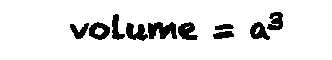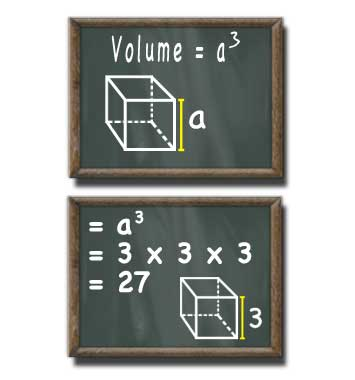# Cube Volume Calculator

## Cube Volume

#### For cubes with a Known Side

Result:
27Side (a) units
decimals

A cube with a side length of 3 units has a volume of 27 cubic units.

This calculator and more easy to use calculators waiting at www.KylesCalculators.com### Calculating the Volume of a Cube:

A cube is a three dimensional shape have equal height, width, and depth. The formula below can be used to find the volume of a cube:

V = a^3

The symbol ^3 means to cube the item preceding it, in this case the side length (a). To cube the side length multiply it by itself twice: a * a * a.

Below is an example of how to find the volume of a cube with the side length of 3.

• V = a^3
• = 3 * 3 * 3
• = 27